#### A potentiometer PQ is set up to compare two resistances as shown in the figure.  The ammeter A in the circuit reads 1.0 A when two way key K3 is open.  The balance point is at a length l1 cm from P when two way key K3 is plugged in between 2 and 1, while the balance point is at a length l2 cm from P when key K3 is plugged in between 3 and 1.  The ratio of two resistancesis  found to be :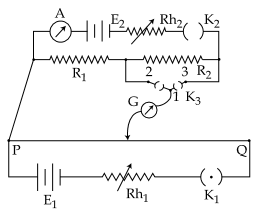Option 1)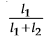Option 2)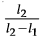Option 3)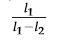Option 4)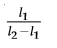As we learnt in

Meter bridge -

To find the resistance of a given wire using a meter bridge and hence determine the specific resistance of its materials

- wherein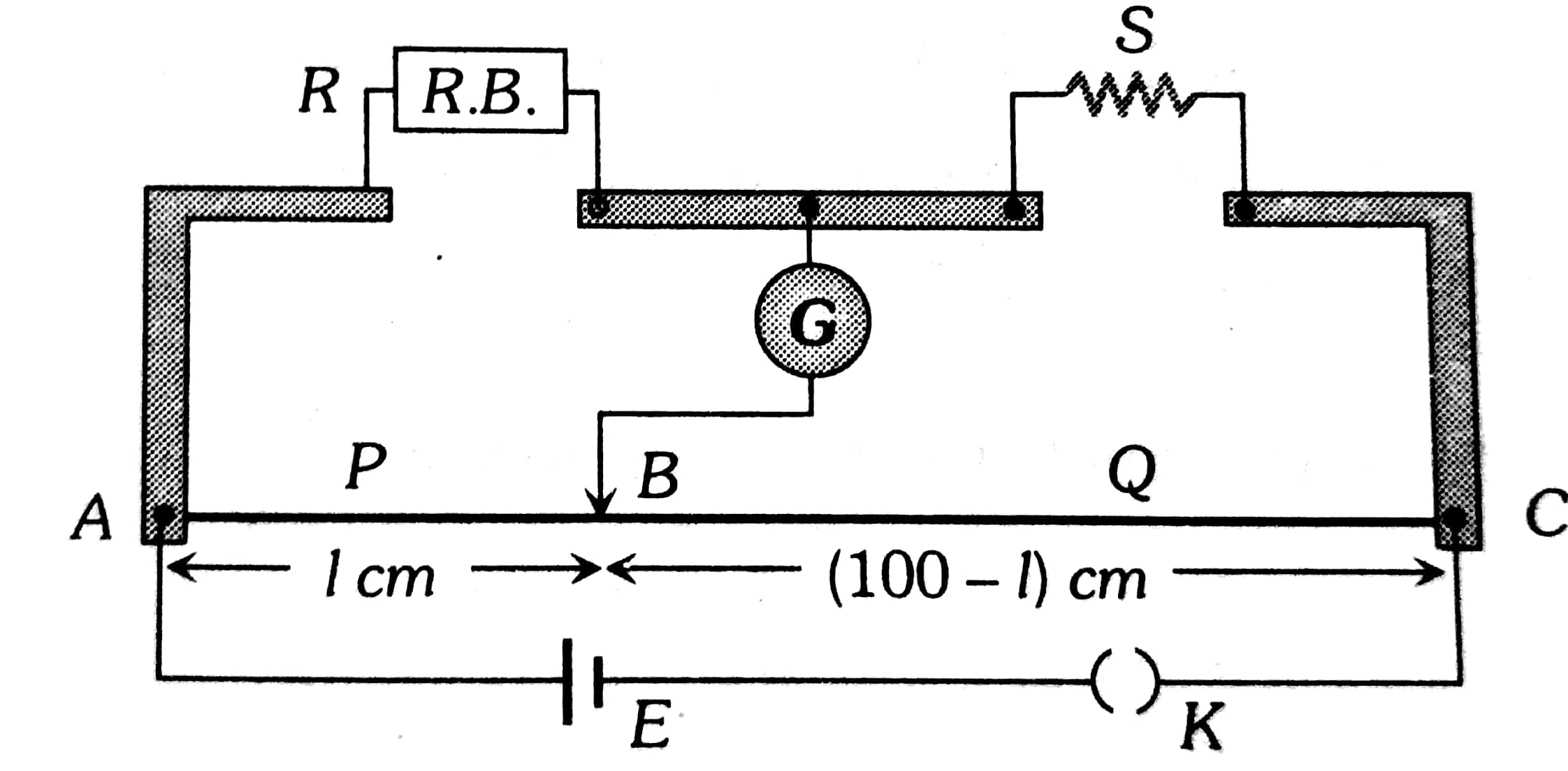When key is at point (1)When key is at 3Option 1)This option is incorrect

Option 2)This option is incorrect

Option 3)This option is incorrect

Option 4)This option is correct

##### Posted by